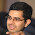## Monday, 13 August 2012

### Varaint of a 10 digit number

Consider a 10-digit number A written as a_1a_2...a_10. A contains each of the digits from 0 to 9 exactly once. The number a_1 is divisible by one. The number a_1a_2 is divisible by two. The number a_1a_2a_3 is divisible by three, and so on...What is A?

#### 1 comment:

1.3816547290

(1) a5 = 5 and a_10 = 0.
(2) The three 3-digit numbers a1a2a3 , a4a5a6 and a7a8a9 must be divisible by 3.
(3) a_i must be have the same parity as 'i'
(4) a3a4 is div by 4 => a4 = 2 or 6 since a3 is odd. Similarly, a6 is even => a7a8 is div by 8 meaning a7a8 can be 16 or 32 or 72 or 96 only. So, a2 = 4 or 8 and a6 = 4 or 8.
(5) a7a8a9 is div by 3 => it can be 321/327/723/729/963. (from (4))

From (5), we get 10 possible numbers. We now need to check for divisibility by 7 for a1a2...a7 .First check the divisibility by 7 for a1a2...a6. Notice that 111111 is divisible by 7 meaning abcdef%7 = (abc-def)%7, where abcdef is a SIX digit number. It can easily be seen that only one number of the ten satisfies.

IF ANYBODY HAS A SIMPLER SOLUTION, PLEASE POST IT.